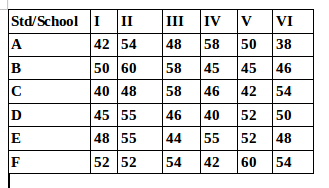## Data Interpretation For SBI PO : Set – 56

D.1-5) Study the table carefully to answer the questions that follow.

Percentage of Students Passing out of six different Colleges in Five years1) If the number of students studying in College B in the year 2005 was 3650, what was the number of students failing from that college in that year?

a) 594

b) 657

c) 583

d) 625

e) None of these

b)

Percentage of students pass from college B in 2005

= 3650*(100-82)/100=3650*18/100=657

2) What is the ratio of the number of students passing to those failing from college E in the year 2002 ?

a) 6:5

b) 18:7

c) 19:6

d) Can’t be determined

e) None of these

c)

Required ratio = 76/100-76 = 76/24 = 19:6

3) If the numbers of students studying in Colleges E and F in the year 2003 were 2800 and 2250 respectively, what was the ratio of the number of students passing out of College E to that out of College F in that year?

a) 216:217

b) 327:341

c) 423:485

d) 539:540

e) None of these

d)

Number of Students Passing out from College E in 2003 = 2800*77/100=28*76

and number of students passing out from College F in 2003 = 2250 *96/100=22.5*96

Required ratio = (28*76/4)/(22.5*96/4)=539/540

4) The average Percentage of Passing is highest for which College?

a) 216:217

b) 327:341

c) 423:485

d) 539:540

e) None of these

e)

By Visual inspection and comparison of given datas it is obvious that percentage of passing is highest for college F

5) What is the percent increase in percentage of students passing out of college A in the year 2005 from the previous year? (Rounded off two digits after decimal)

a) 7.61

b) 8.24

c) 6.36

d) 8.05

e) None of these

b)

Required Percentage increase = (92- 85/85)*100=7/85*100=8.235=8.24

D.6-10) Study the table carefully to answer the questions that follow.

Number of students studying in different standards of six different schools6) What is the approximate average number of students studying in Standard I from all schools together?

a) 38

b) 50

c) 40

d) 43

e) 46

e)

Average number of students studying in standard I from all school together

42+50+40+45+48+52/6= 277/6=46.16=46

7) Number of students in standard IV from school E is What percent of those studying in standard IV from School D?

a) 128

b) 132.5

c) 124

d) 137.5

e) None of these

d)

Required Percentage = 55*100 /40 = 137.5

8) Which Standard has the lowest total number of students from all the given schools together?

a) V

b) VI

C) I

d) IV

e) None of these

c)

Total number of students in Standard I = 277

Total number of students in Standard II = 324

Total number of students in Standard III = 308

Total number of students in Standard IV = 286

Total number of students in Standard V = 301

Total number of students in Standard VI = 290

So the lowest number of students is in Standard I

9) Which School has the highest total number of students from all the given standards together?

a) E

b) F

c) B

d) A

e) None of these

b)

Total number of students in School A = 290

Total number of students in School B = 304

Total number of students in School C = 288

Total number of students in School D = 288

Total number of students in School E = 302

Total number of students in School F = 314

So total number of students from the given standards together is highest in School F

10) what is the respective ratio of students studying in Standard III of schools A and B together to those studying in Standard VI of schools C and D together?

a) 53:52

b) 43:47

c) 25:27

d) 39:38

e) None of these